Courses

# Short Answer Type Questions- Areas Related to Circles Class 10 Notes | EduRev

## Class 10 : Short Answer Type Questions- Areas Related to Circles Class 10 Notes | EduRev

The document Short Answer Type Questions- Areas Related to Circles Class 10 Notes | EduRev is a part of the Class 10 Course Mathematics (Maths) Class 10.
All you need of Class 10 at this link: Class 10

Q1. In a circular table cover of radius 70 cm, a design is formed leaving an equilateral triangle ABC in the middle as shown in the figure. Find the total area of the design.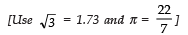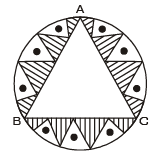Sol. ∵ Radius of the circle = 70 cm and O is the centre of the circle.
∴ OA = OB = OC
Since ∆ABC is an equilateral triangle,
∴∠ABO = ∠BOC= ∠CDA = 120°
Draw OD⊥BC
Now in right ΔBDO,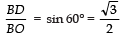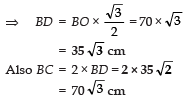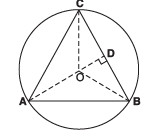∴ Area of equilateral ΔABC =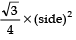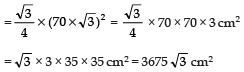Also, area of the circle = πr2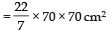= 22 × 10 × 70 cm2 = 15400 cm2

∴ Area of the shaded region
= 15400 cm2 − (3675√3) cm
= 15400 cm2 − (3675 × 1.73) cm2
= 15400 cm2 − 6357.75 cm2 = 9042.25 cm2.

Q2. Calculate the area other than the area common between two quadrants of the circles of radius 16 cm each, which is shown as the shaded region in the figure.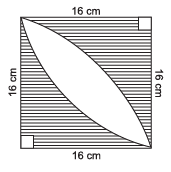Sol.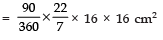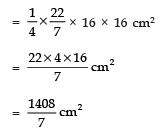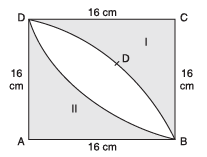∴ Area of the shaded region-I =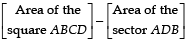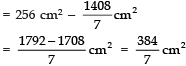Similarly, in area of the shaded region-II 384/7 cm2

∴ Total area of the shaded region = [Area of shaded region-I] + [Area of shaded region-II]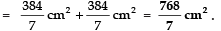Q3. In the figure, PQ = 24 cm, PR = 7 cm and O is the centre of the circle. Find the area of shaded region. (Take π = 3.14)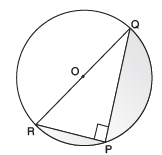Sol. In right Δ RPQ,
PR2 + PQ2 = RQ2
⇒ 72 + 242 = RQ2
⇒ 49 + 576 = RQ2
⇒ 625 = RQ2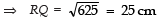⇒ Radius of semi-circle = 25/2 cm

∴ Area of semi-circle RQP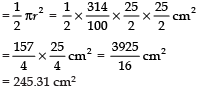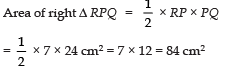∴ Area of the shaded region=Area of semi-circle RQP − ar (right Δ RPQ)
=245.31 cm2 = 84 cm2 = 161.31 cm2.

Q4. The area of an equilateral triangle is 49√3. Taking each angular point as centre, circles are drawn with radius equal to half length of the side of the triangle. Find the area of triangle not included in the circles. [Take √3 = 1.73]

Sol. Let the given equilateral triangle be ABC, such that its side = 14 cm.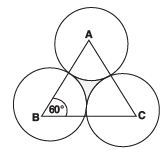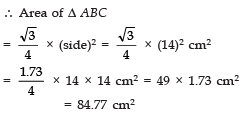Since each angle of an equilateral triangle = 60°,
∴ Area of a sector having θ as 60° and radius 14/2 i.e. 7 cm.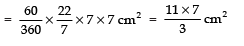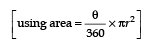⇒ Area of 3 sectors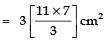= 77 cm2
∴ Area of the shaded region = [Area of equilateral ∆ ABC] − [Area of 3 sectors]
= 84.77 cm2 − 77 cm2 = 7.77 cm2.

Q5. Four equal circles are described at the four corners of the square so that each touches two of the others, the shaded area enclosed between the circles is 22/7 cm2 Find the radius of each circle.

Sol. Let 'r' cm be the radius of each circle.
∴ side of the square = 2r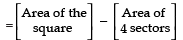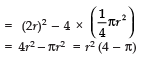But the area of shaded region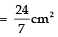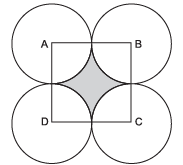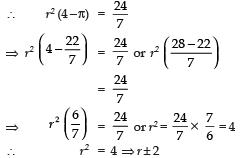But r cannot be − ve, so r = 2 cm

Q6. A square OABC is inscribed in a quadrant OABQ of a circle as shown in the figure. If OA = 14 cm, find the area of the shaded region. [use π = 22/7]

Sol. OABC is a square with side = 14 cm.
∴ Area of the square OABC = 14 cm × 14 cm = 196 cm2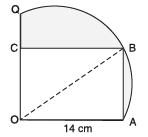Now, the diagonal of the square OABC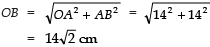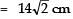∴ Area of the quadrant OABQ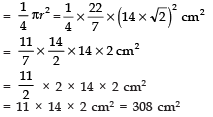∴ Area of the shaded region
= 308 cm2 − 196 cm2 = 112 cm2.

Q7. In the figure, ABDC is a quadrant of a circle of radius 14 cm and a semi-circle is drawn with diameter BC. Find the area of the shaded region.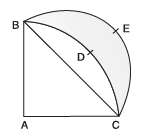Sol. We have, in the right Δ ABC,

BC2 = AB2 + AC2 = 142 + 142 = 2 (14)2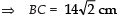∴ Radius of the semi-circle =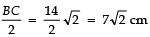∴ Area of semi-circle BEC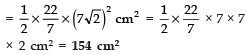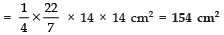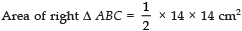= 98 cm2

Area of the shaded region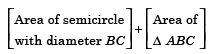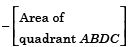= 154 cm2 + 98 cm2 − 154 cm2 = 98 cm2.

Q8. In the figure, find the perimeter of the shaded region where, ADC, AEB and BFC are semicircles on diameters AC, AB and BC respectively.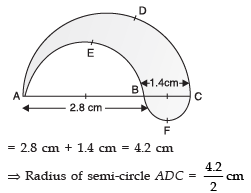= 2.1 cm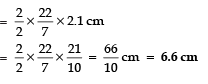Diameter of semi-circle AEB = 2.8 cm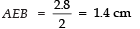∴ Circumference of semi-circle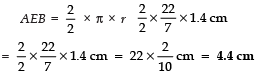Diameter of semi-circle BFC = 1.4 cm
⇒ Radius of semi-circle BFC = 0.7 cm
∴ Circumference of semi-circle BFC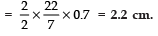∴ Total perimeter of the shaded region = 6.6 cm + 4.4 cm + 2.2 cm = 13.2 cm.

Q9. In the figure, arcs are drawn by taking vertices A, B and C of an equilateral triangle of side 10 cm to intersect the sides BC, CA and AB at their respective mid-points D, E and F. Find the area of the shaded region.

[use π = 3.14]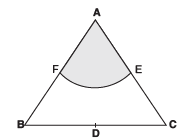Sol. ∵ ΔABC is an equilateral triangle.
∴∠A = ∠B = ∠C = 60°
Area of sector AFEA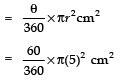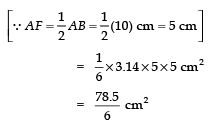Area of all the three sectors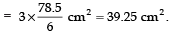Thus, area of the shaded region = 39.25 cm2.

Q10. In figure OABC is a quadrant of a circle of radius 7 cm. If OD = 4 cm, find the area of the shaded region. [Use π = 22/7]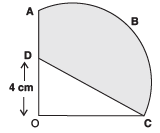Sol. We have, the centre of the circle as ‘O’ and radius (r) = 7 cm

Area of the quadrant OABC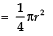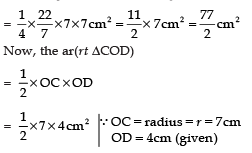=  7 × 2 = 14 cm2
∴ Area of the shaded region = (Area of the quadrant OABC) – (Area of ∆COD)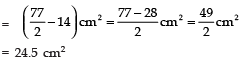Offer running on EduRev: Apply code STAYHOME200 to get INR 200 off on our premium plan EduRev Infinity!

## Mathematics (Maths) Class 10

178 videos|268 docs|103 tests

,

,

,

,

,

,

,

,

,

,

,

,

,

,

,

,

,

,

,

,

,

;